• 50
•
• 3
•
•
•
•
•
53
Shares

# OHM’S LAW : BASICS

If you think about electrical physics then you will find that in electrical physics there is only three basic things i.e voltage, current and resistance, without these three things study of electrical physics seems meaningless.

These three things are the basic quantity in electrical physics. If anyone wants to study electricity in detail and deeper then they should first go through by these three quantity i.e voltage, current and resistance.

They should first understand these three quantities and should know that how these three quantities are related to each other. In this article we are going to discuss about the relationship between these three quantities in detail, so stay tuned us with us.

In 1827 a treatise was published by German physicist Georg Simon Ohm. In this treatise, he had explained that if we take a circuit of fixed length and then if we supply different different voltage in the same circuit then we get different different value of electric current.

He tells that value of electric current rises in the circuit as we increase the voltage. Means, electric current is directly proportional to the voltage. This statement changes the study of electrical physics completely and give a new way to understand and development in electrical engineering.

He further tells that in every circuit there is something which opposes the flow of motion of electric charges. He termed this opposing nature of circuit as resistance.

This ground breaking statement further become a law called ohm’s Law.

## OHM’S LAW : STATEMENT

Ohm’s law states that the current through a conductor between two points is directly proportional to the voltage across the two points, provided that physical condition are kept constant.

If current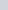is flowing through a circuit due to a potential difference (V) across the terminal of the circuit. Then, mathematically –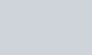Where R is the resistance of the circuit. It’s value depends upon the length, cross sectional area and shape and the nature of the material of the conductor.

## DEDUCTION OF OHM’S LAW

In the previous article we have derived the formula for drift velocity. Now, we will deduce the ohm’s Law from the formula of drift velocity.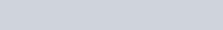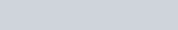This is ohm’s Law.

## HYDRAULIC ANALOGUE OF OHM’S LAW

### WHAT IS ANALOGY?

There are various analogy of ohm’s Law in Nature, but the analogy we know is hydraulic analogy. In this section we will understand how ohm’s Law is analogy to water flow.

First of all let’s understand what is analogy.

Analogy is the way of comparison based on how two things are alike.

For example – here we are giving two important formula of the same kind.
Formula of gravitational force between two masses.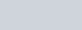And formula of electrostatic force between two points charges.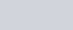Here you can clearly see that these two formula are of the same kind only quantities are changed.

So we can say these two formula are analogy. Hope you got understood.

### HYDRAULIC ANALOGY

Did you know?, how does water flow in a pipe?. If you live in villages then you must have seen water flowing inside the pipe from the submersible pumps during irrigating the crops field.

Now let’s come on the topic. How did water inside the pipe going ahead? Water is flowing ahead because of pressure difference at the both end of the pipe.

The end which is at the submersible pump have the high pressure because at this point water is coming out and the second end which is in the crop field have the lowest pressure. This pressure difference tends water to flow ahead.

1). This pressure difference is analogy to potential difference (V) because potential difference also tends electrons to flow from higher potential to lower potential.

2). Water which are flowing inside the pipe at the speed of litre per second is the analogy of electric current coulomb per second inside the conductor.

3). Roughness and apertures inside the pipe acts as restrictor which minimises the flow speed of the water. This restrictors are the analogy to the resistance of the conductor which minimises the flow rate of the electrons.

So, we see that water flowing through any pipe can be described as ohm’s Law. Flow and pressure difference can be calculated in fluid flow network with the use of the hydraulic ohm analogy. This method can be applied to both steady and transient flow situations.

## OHM’S LAW MAGIC TRIANGLE

If you want to remember the different formulae related to ohm’s Law. Then for this, there is a triangle called ohm’s Law magic triangle. This triangle is said to be magical because it makes remembering very easy. After knowing this triangle you will only say that’s awesome and Why this triangle aren’t in our physics textbook?.

The triangle which we are talking about is just a triangle in which topmost corner of the triangle is letter and left and right of bottom corner is letter I and R,  see figure below :

### HOW TO USE THIS TRIANGLE

If you want to find the value of V then cover this V letter with your finger or palm and the remaining letter give the value of the covered letter, i.e V =I×R.

And if you want to find the value of I then cover the letter I with your finger or palm and the remaining letter give the required value, i.e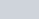.

And similarly if you want to find the value of R then cover it and then remaining letter will be your answer. i.e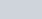. See figure below :

## EXPERIMENTAL VERIFICATION OF OHM’S LAW

We know that, currentflowing through any circuit is directly proportional to the potential difference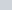across the circuit. It can be written as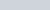, where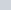is the proportionality constant called resistance of the conductor. This is ohm’s Law.

This ohm’s Law can be verified in the lab or at home very easily. You only have to make some basic setup for which you will need following materials.

MATERIAL REQUIRED

• Voltmeter
• Ammeter
• Power source (dry cells)
• Plug keys
• Some connecting wires

PROCEDURE

1. Take four or five dry cells, voltmeter, ammeter, plug keys and thick connecting wires. Join these materials as shown in the following figure.Experimental verification of ohm’s Law, image source : concepts-of-physics

2. Start your experiment with single cell as a power source. Key plug is used when electric current is not required. While using single cell in the circuit as power source then note the reading in the voltmeter as well ammeter in your notebook.
3. Now use two cell in circuit as power source, now see the reading of the voltmeter and ammeter and record the respective reading in your notebook.
4. Perform this activity similarly with three, four and five cells/battery one by one and keep recording the reading in case case.
5. Now draw a graph between V and I on the graph paper. You will see a approximately straight line from the origin. This straight line show that I is directly proportional to the voltage across the circuit i.e ohm’s Law.

6. For every value of V and I there is fixed value of R. Means R remain constant in whole experiment.

## LIMITATIONS OF OHM’S LAW

On the basis of ohm’s Law there are many electrical materials which obeys the ohm’s Law and many others do not obeys ohm’s Law.

The materials which obeys the ohm’s Law their resistance didn’t changes if there current and voltage changes such materials are called ohmic materials. They have linear relationship between voltage and current. Due to this ohmic materials may also be called linear electronic components.

But there also such many materials called non ohmic materials which didn’t obeys the ohm’s Law and their resistance also changes if their voltage and current changes. Such non ohmic materials may also called non-linear as they do not have a linear relationship between voltage and current.

### SOME LIMITATIONS OF OHM’S LAW

Some limitations are listed below :

1. Ohm’s law cannot be applied to unilateral networks. A unilateral network has unilateral elements like diode, transistors, etc., which do not have same voltage current relation for both directions of current.
2. Ohm’s law is not applicable for non – linear components. Non-linear components are those which do not have current exactly proportional to the applied voltage, that means the resistance value of those elements changes for different values of voltage and current. Examples of non – linear elements are thyristor, electric arc, etc.

A interactive visualisation of ohm’s Law in a electric circuit given below. This visualisation helps you to understand how current, voltage and resistance are related to each other. Play and enjoy the visualisation.